# Tag Archives : #FebruaryTeacherTalk

## February Teacher Talk

Posted by Deann Marin of Socrates Lantern

Welcome to our February Teacher Talk.  All of us from the Teacher Talk collaborative would like wish you a Happy Valentines Day.  We have so many fab things this month from  proof-reading ideas, to ELA to math activities, to celebrating Black History Month and President’s Day, you don’t want to miss reading these blog posts from some awesome educators. This month, we’re honored to have Daniel with us, as our  guest blogger.
If you’re interested in joining this unique group of teacher entrepreneurs and blogging buddies and our blog linky, sign up here…. If you decide to join, be sure to mention one of our names.
<<<<<<<<<<<<<<<<<<<<<<<<<<<<<<<<<<<<<<<<<<<<<<<<<<<<<<<<<<<
Navigating Life as a Single Dad
By Daniel of Dad Solo whose guest post can be found at Rainbow City Learning

Single parents have a difficult job, and even in the best of circumstances, it can be a struggle. Moms have it tough, but so do dads.
<<<<<<<<<<<<<<<<<<<<<<<<<<<<<<<<<<<<<<<<<<<<<<<<<<<<<<<<<<<
I Dreaded my Third Period Class
By Deann Marin of Socrates Lantern

It was cold and blustery outside, one of those days that you just wanted to stay under the covers. But it was only hump day and I had a busy schedule, so I dragged myself out of bed, had my cup of coffee and a piece of toast, jumped into my car and was on my way.
<<<<<<<<<<<<<<<<<<<<<<<<<<<<<<<<<<<<<<<<<<<<<<<<<<<<<<<<<<<

Is This Routine? Why Not?
By Susan Berkowitz of Susan Berkowitz

Research tells us that routines are the heart of language and symbbol development. Routines are a sequence of actions or events that are repeated over and over again.
<<<<<<<<<<<<<<<<<<<<<<<<<<<<<<<<<<<<<<<<<<<<<<<<<<<<<<<<<<<

By Sally Hansen of Purposeful Plans

Have you ever set a goal to cover everything you are required to teach during the school year?  Many teachers try to meet this goal each year and end up frustrated because we are short on time!  It is very difficult to get everything we are required to teach during the school year, take the cross-curricular approach!
<<<<<<<<<<<<<<<<<<<<<<<<<<<<<<<<<<<<<<<<<<<<<<<<<<<<<<<<<<<

Improving Class Cooperation
By Gini Musmanno of Reading Spotlight

Wishing and hoping, and thinking and praying, for a courteous and amenable class? Then give students a little nudge toward cooperation and affirmation of each other this month.
<<<<<<<<<<<<<<<<<<<<<<<<<<<<<<<<<<<<<<<<<<<<<<<<<<<<<<<<<<<

By Lisa Robles of LisaTeachR’s Classroom

<<<<<<<<<<<<<<<<<<<<<<<<<<<<<<<<<<<<<<<<<<<<<<<<<<<<<<<<<<<

Building Understanding, One Brick at a Time
By Kathie Yonemura of Tried & True Teaching Tools

Getting your hands muddy is a great way to learn! Social Studies is meaningful when students experience content learned.
<<<<<<<<<<<<<<<<<<<<<<<<<<<<<<<<<<<<<<<<<<<<<<<<<<<<<<<<<<<

How to Select Books for Your Classroom Book Clubs
By Marcy Howe of It’s a Teacher Thing

Read five essential tips for finding “just right” books for your classroom book clubs. The books you choose are crucial to running successful classroom book clubs.
<<<<<<<<<<<<<<<<<<<<<<<<<<<<<<<<<<<<<<<<<<<<<<<<<<<<<<<<<<<
By Thia Triggs of Print Path

What does research tell us about the impact of fine motor skills on early academics?
<<<<<<<<<<<<<<<<<<<<<<<<<<<<<<<<<<<<<<<<<<<<<<<<<<<<<<<<<<<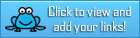## February Teacher Talk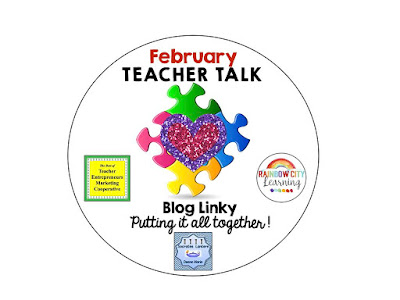Valentine Day, President’s Day, Black History Month, Kindness Week and so many teacher tried and true ideas are here for you this February. So pull up a chair sip some hot chocolate and savor some Valentine goodies will reading our englightening blog posts.

If you’re interested in joining this unique group of teacher entrepreneurs, blogging buddies and/or our blog linky, sign up here….The Best of Teacher Entrepreneurs Marketing Cooperative. If you decide to join, be sure to mention one of our names.

***********************************************

Light up the World with Kindness

By Deann Marin of Socrates LanternDid you know that there is a Random Acts of Kindness Week? It runs from February 12 through the 18th. Actually, this is the first I’ve heard of it, but what a groovy  way for all of us to unite by being kind to each other.

****************************************************

Make Math FUN this Valentine’s Day!

By Sara Snyder of Snyder ClassroomValentine’s Day can be a fun time of year! It can a busy time and also little distracting. I have often found myself looking for fun yet engaging activities for my students to use while still sticking with my curriculum. I have made these math riddles to combine fun and learning for this time of year.

*************************************************

AAC 101: Who Should Use AAC and Why?

By Susan Berkowitz of Susan BerkowitzAnyone who does not have speech or whose speech is not sufficient to meet their needs should be considered for an AAC system.

*************************************************

By Thia Triggs of Print PathWhether you are a parent, teacher, or therapist, do you know children who would benefit from play experiences and practice performing tasks that do not come easily?

************************************************

The Broken-Winged Bird: Musings on Poetry and Complex Text

By Tracy Willis of Wild Child DesignsWade into the deep end and use poetry in your reader’s workshop. This post lays out several strategies to deepen your students’ reading comprehension when using poetry. Explore compare/contrast, figurative language, character theory, and metaphorical thinking with Wild Child Designs.

***********************************************

Use Your Literature Text to Teach History

By Marcy Howe of It’s a Teacher ThingSometimes I find exactly what I need in the most unlikely place. My literature anthology recently proved to be the perfect vehicle for teaching historical context, and the lesson went better than I could have imagined!

**************************************************

Be sure to read the posts from the rest of the teachers in this month’s post link up.## February Teacher Talk

Posted by Deann Marin of Socrates Lantern

Welcome to our February Teacher Talk.  All of us from the Teacher Talk collaborative would like wish you a Happy Valentines Day.  We have so many fab things this month from  proof-reading ideas, to ELA to math activities, to celebrating Black History Month and President’s Day, you don’t want to miss reading these blog posts from some awesome educators.If you’re interested in joining this unique group of teacher entrepreneurs and blogging buddies and our blog linky, sign up here….The Best of Teacher Entrepreneurs Marketing Cooperative. If you decide to join, be sure to mention one of our names.

<<<<<<<<<<<<<<<<<<<<<<<<<<<<<<<<<<<<<<<<<<<<<<<<<<<<<<<<<<<

The Proof is in the Pudding, Proof-Reading That Is!As a veteran English and Social Studies teacher, I’d like to share some effective proof-reading tips that I’ve used to help students improve their writing skills, and to make my life a bit easier.

<<<<<<<<<<<<<<<<<<<<<<<<<<<<<<<<<<<<<<<<<<<<<<<<<<<<<<<<<<<

Love is All We Need

By Retta London of Rainbow City LearningJust a little inspiration for building confidence and acceptance in your learning community.

<<<<<<<<<<<<<<<<<<<<<<<<<<<<<<<<<<<<<<<<<<<<<<<<<<<<<<<<<<<

IMWAYR: Funny Bones

By Lisa Robles of LisaTeachR’s ClassroomHave you read Funny Bones? Winner of multiple awards and a great informational addition to your Dia de Los Muertos collection!

<<<<<<<<<<<<<<<<<<<<<<<<<<<<<<<<<<<<<<<<<<<<<<<<<<<<<<<<<<<

Valentine’s Day STEM Olympics

By Kerry Tracy of Kerry TracyBlog post outlines 5 infatuating Valentine’s Day-themed STEM challenges that can be modified for use with grades 2-8. Help Cupid get some target practice, build a tower of love, design the perfect candy container, find the “heaviest” heart, and have a flower frenzy!

<<<<<<<<<<<<<<<<<<<<<<<<<<<<<<<<<<<<<<<<<<<<<<<<<<<<<<<<<<<

Cooperative Learning – Bring Core Subjects Together for Student Learning!

By M. Moore of Moore ResourcesCooperative Learning – Bring Core Subjects Together for Student Learning!

<<<<<<<<<<<<<<<<<<<<<<<<<<<<<<<<<<<<<<<<<<<<<<<<<<<<<<<<<<<

What Word Does This Say: B-L-E-N-D?

By Susan Berkowitz of Susan BerkowitzThis is part of a series of posts about phonological awareness and the different steps and skills to teach. This post is about blending sounds and syllables to hear the word.

<<<<<<<<<<<<<<<<<<<<<<<<<<<<<<<<<<<<<<<<<<<<<<<<<<<<<<<<<<<

Teaching Self-Regulation Skills to Elementary Age Children

By Thia Triggs of Print PathFinally! A systematic method to teach self-regulation skills to children with sensory, emotional, and behavioral needs. Color coded task cards give students managed choices to learn specific skills that meet their sensory needs and also calm them down, so that they can perform their academic classroom tasks.

<<<<<<<<<<<<<<<<<<<<<<<<<<<<<<<<<<<<<<<<<<<<<<<<<<<<<<<<<<<

My Funny Valentine: Love Letters by Arnold Adoff

By Tracy Willis of Wild Child Designs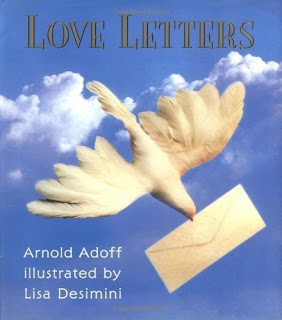A review and glimpse into “Love Letters” poetry by Arnold Adoff. This post also includes a teaching idea for its use in the classroom.

<<<<<<<<<<<<<<<<<<<<<<<<<<<<<<<<<<<<<<<<<<<<<<<<<<<<<<<<<<<

Robots + Math & Science = Total Engagement

By Megan Bodmann of Adventures Teaching 4thGet your students engaged in learning by introducing robots into your classroom. They are not only a ton of fun, but you can easily utilize them in your math and science lessons. Find out how easy it is to do!

<<<<<<<<<<<<<<<<<<<<<<<<<<<<<<<<<<<<<<<<<<<<<<<<<<<<<<<<<<<

Playground Problem = Real Life

By Kathie Yonemura of Tried and True Teaching Tools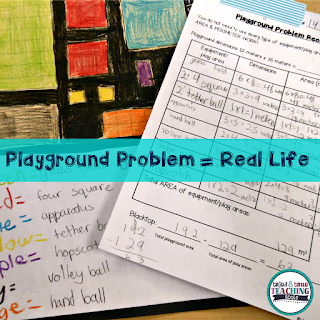The Playground Problem is a real-life math challenge! It keep students engaged and practicing their area and perimeter skills in a meaningful way.

<<<<<<<<<<<<<<<<<<<<<<<<<<<<<<<<<<<<<<<<<<<<<<<<<<<<<<<<<<<

Understanding Fractions: A 6-Part Series

By Shametria Routt of The Routty Math TeacherFractions– a single word that deflates the confidence of our most competent students and adults alike. In this series, I share some of the essential fraction understandings that I have developed over the years, including the many math tools you can use to reinforce these essential skills. With that in mind, each of the six posts highlights a fraction tool and activity that can be used to address a specific Common Core Math Standard (with a few printable freebies too).

<<<<<<<<<<<<<<<<<<<<<<<<<<<<<<<<<<<<<<<<<<<<<<<<<<<<<<<<<<<

Leveled Math Assessments

By Tammy Roose of Tarheel State Teacher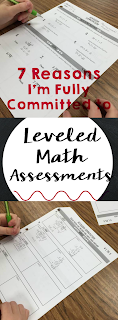Do your math assessments encourage a growth mindset? Create an entry point for students who are not yet meeting the standards? Allow above average learners to show they know more than just what’s expected for their grade-level? Find out 7 reasons why I’m committed to leveled math assessments this year!

<<<<<<<<<<<<<<<<<<<<<<<<<<<<<<<<<<<<<<<<<<<<<<<<<<<<<<<<<<<

Here’s your chance to hop on over and visit the blog posts of our creative teachers.